Next: Eigenvalues of Up: Orbital Angular Momentum Previous: Representation of Angular Momentum

Eigenstates of Angular Momentum

Let us find the simultaneous eigenstates of the angular momentum operatorsand. Since both of these operators can be represented as purely angular differential operators, it stands to reason that their eigenstates only depend on the angular coordinatesand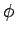. Thus, we can write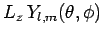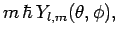(556)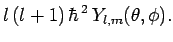(557)

Here, the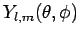are the eigenstates in question, whereas the dimensionless quantitiesandparameterize the eigenvalues ofand, which are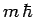and, respectively. Of course, we expect theto be both mutually orthogonal and properly normalized (see Sect. 4.9), so that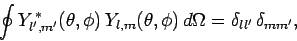(558)

where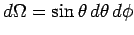is an element of solid angle, and the integral is over all solid angle.

Now,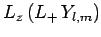(559)

where use has been made of Eq. (543). We, thus, conclude that when the operator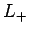operates on an eigenstate ofcorresponding to the eigenvalueit converts it to an eigenstate corresponding to the eigenvalue. Hence,is known as the raising operator (for). It is also easily demonstrated that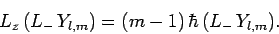(560)

In other words, whenoperates on an eigenstate ofcorresponding to the eigenvalueit converts it to an eigenstate corresponding to the eigenvalue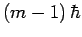. Hence,is known as the lowering operator (for).

Writing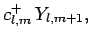(561)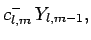(562)

we obtain(563)

where use has been made of Eq. (541). Likewise,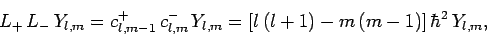(564)

where use has been made of Eq. (540). It follows that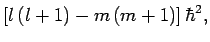(565)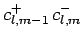(566)

These equations are satisfied when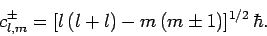(567)

Hence, we can write(568)(569)Next: Eigenvalues of Up: Orbital Angular Momentum Previous: Representation of Angular Momentum
Richard Fitzpatrick 2010-07-20